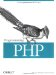# 5.9 Acting on Entire Arrays

PHP has several useful functions for modifying or applying an operation to all elements of an array. You can merge arrays, find the difference, calculate the total, and more, all using built-in functions.

#### 5.9.1 Calculating the Sum of an Array

The array_sum( ) function adds up the values in an indexed or associative array:

`\$sum = array_sum(array);`

For example:

`\$scores  = array(98, 76, 56, 80); \$total   = array_sum(\$scores); // \$total = 310`

#### 5.9.2 Merging Two Arrays

The array_merge( ) function intelligently merges two or more arrays:

`\$merged = array_merge(array1, array2 [, array ... ])`

If a numeric key from an earlier array is repeated, the value from the later array is assigned a new numeric key:

`\$first  = array('hello', 'world');      // 0 => 'hello', 1 => 'world' \$second = array('exit',  'here');       // 0 => 'exit',  1 => 'here' \$merged = array_merge(\$first, \$second); // \$merged = array('hello', 'world', 'exit', 'here')`

If a string key from an earlier array is repeated, the earlier value is replaced by the later value:

`\$first  = array('bill' => 'clinton', 'tony' => 'danza'); \$second = array('bill' => 'gates',   'adam' => 'west'); \$merged = array_merge(\$first, \$second); // \$merged = array('bill' => 'gates', 'tony' => 'danza', 'adam' => 'west')`

#### 5.9.3 Calculating the Difference Between Two Arrays

The array_diff( ) function identifies values from one array that are not present in others:

`\$diff = array_diff(array1, array2 [, array ... ]);`

For example:

`\$a1 = array('bill', 'claire', 'elle', 'simon', 'judy'); \$a2 = array('jack', 'claire', 'toni'); \$a3 = array('elle', 'simon',  'garfunkel'); // find values of \$a1 not in \$a2 or \$a3 \$diff = array_diff(\$a1, \$a2, \$a3); // \$diff is array('bill', 'judy');`

Values are compared using ===, so 1 and "1" are considered different. The keys of the first array are preserved, so in \$diff the key of 'bill' is 0 and the key of 'judy' is 4.

#### 5.9.4 Filtering Elements from an Array

To identify a subset of an array based on its values, use the array_filter( ) function:

`\$filtered = array_filter(array, callback);`

Each value of array is passed to the function named in callback. The returned array contains only those elements of the original array for which the function returns a true value. For example:

`function is_odd (\$element) {   return \$element % 2; } \$numbers = array(9, 23, 24, 27); \$odds    = array_filter(\$numbers, 'is_odd'); // \$odds is array(0 => 9, 1 => 23, 3 => 27)`

As you see, the keys are preserved. This function is most useful with associative arrays.Programming PHP
ISBN: 1565926102
EAN: 2147483647
Year: 2007
Pages: 168

Similar book on Amazon